### Home > CAAC > Chapter 4 > Lesson 4.1.7 > Problem4-62

4-62.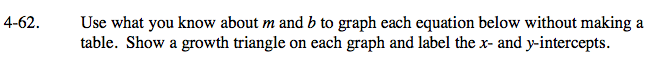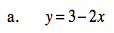Identify m and b.

m = −2, b = 3

Graph b, the y-intercept.

Use m to draw the rest of the line.

x-intercept = (1.5, 0)
y-intercept = (0, 3)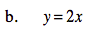This is equivalent to y = 2x + 0.
Follow the steps in part (a).

x-intercept = (0, 0) y-intercept = (0, 0)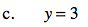This is equivalent to y = 0x+3.
Follow the steps in part (a).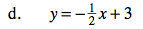Follow the steps in part (a).

Use the eTool below to graph the equations without making a table.
Click the link at right for the full version of the eTool: AC 4-62 HW eTool